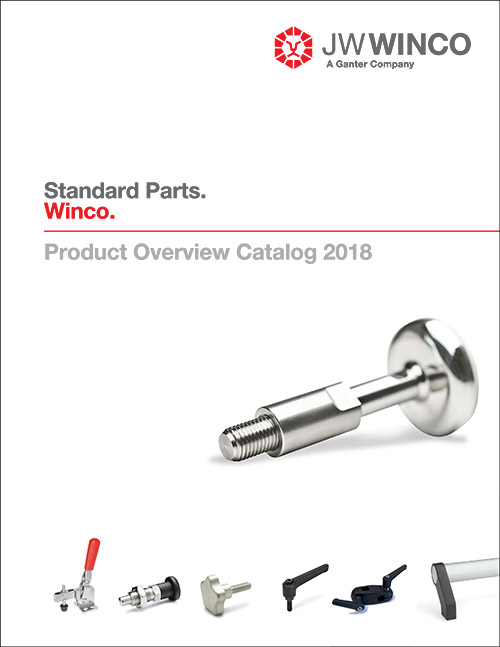Friction Bearings Determining the Size | J.W. Winco Piezas Estándar

# Determining the Size of Universal Joints with Friction Bearings

## DIN 808-FB

The graph shows the transmitted outputs "N" or torques "M" of universal joints as defined in DIN 808-FB (single, friction bearing), depending on the speed "n."   This information is applicable for uniform speed, uniform load and a maximum working angle of 10°. It is not applicable for stainless steel universal joints.

Where the working angles "ß" are larger, an assumed reference output "N" or an assumed torque "M," increased by correction factor "k" must be used (see example below).

Conversion formulas:

Torque M [Nm] = 9550 N [kW] / n [rpm]
Output N [kW]= M [Nm] x n [rpm]
1 kW = 1.36 hp     1 hp = 0.736 kW

Example 1:

Transmitted output N = 0.65 kW
Speed n= 230 rpm Working angle ß= 10°
Correction factor k= 1

Reference output "N" = Rated Output N Intersection "P" is derived from 0.65 kW and 230 rpm (corresponding to a torque of 27 Nm).

The next larger joint corres-ponding to Point P is d1 = 25

Example 2:

Transmitted torque M = 27
Nm Speed n= 230 rpm
Working angle ß= 30°
Correction factor k= 2.25
Reference torque M = 2.25 x 27 Nm = 61
Nm Intersection "P1" is derived from 61 Nm and 230 rpm (corresponding to a reference output "N" = 1.44 kW).
The next larger joint corres-ponding to Point P1 is d1 = 32

Hinweis zur Weiterleitung

Für diese Landesversion verlassen Sie die aktuelle Webseite und werden zur jeweiligen Landesvertretung weitergeleitet. Bitte beachten Sie, dass es zu Abweichungen von Darstellung und Inhalten kommen kann.

Sie werden nicht automatisch weitergeleitet. Bitte klicken Sie den unten genannten Link.

For this country version you leave the current website and are forwarded to the respective country representation. Please note that there may be deviations in presentation and content.

You will not be redirected automatically. Please click on the link below.

Cerca
JW Winco - Contact

United States
E-Mail: sales@jwwinco.com
Phone: 800-877-8351
Fax: 800-472-0670
Hours M-F 7:30 AM - 5:00 PM CT